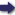(London :  Kegan Paul, Trench, Trübner & Co.,  1910.)

 Tools

## Search this bookPrev Page 58 Next``` 58 ALBERUNTS INDIA. Method of Pulisa for the same purpose. Explana¬ tory notes thereon. This kind of computation may be continued if we want to have seconds and minor values. The quotient represents the place of that planet according to its mean motion, or the place of that apsis or that node which we wanted to find. The same is also mentioned by Pulisa, but his method differs, as follows:—" After having found the com]3lete cycles which have elapsed at a cer¬ tain moment of time, he divides the remainder by 131,493,150. The quotient represents the mean signs of the ecliptic. "The remainder is divided by 4,383,105. The quo¬ tient represents degrees. The fourfold of the remainder is divided by 292,207. The quotient represents minutes. The remainder is multiplied by 60 and the product divided by the last-mentioned divisor. The quotient represents seconds. " This calculation may be continued, so as to give third parts, fourth parts, and minor values. The quo¬ tient thus found is the mean place of the planet which we want to find." The fact is that Pulisa was obliged to multiply the remainder of the cycles by 12, and to divide the pro-^ duct by the days of a cctturyugct, because his whole computation is based on the cctturyuga. But instead of doing this, he divided by the quotient which you get if you divide the number of days of a caturyuga by 12. This quotient is the first number he mentions, viz. 131,493,150. Further, he was obliged to multiply the remainder of the signs of the ecliptic by 30, and to divide the product by the first divisor; but instead of doing this, he divided by the quotient which you get if you divide the first number by 30. This quotient is the second number, viz. 4,383,105. According to the same analogy, he wanted to divide the remainder of the degrees by the quotient which ```Prev Page 58 Next